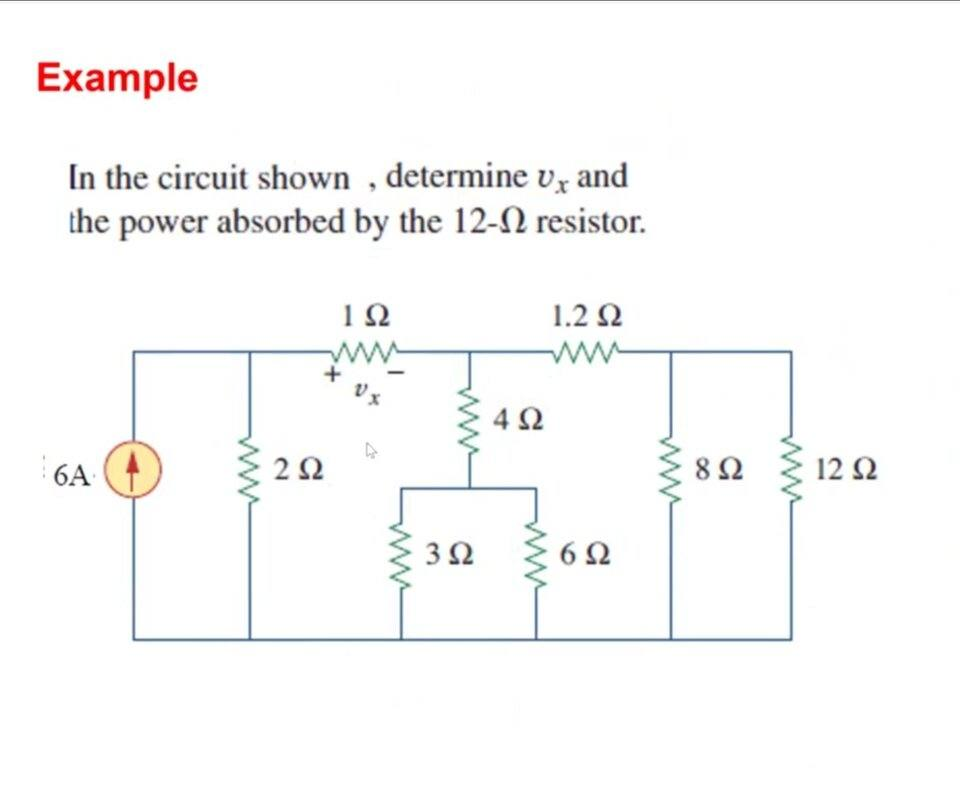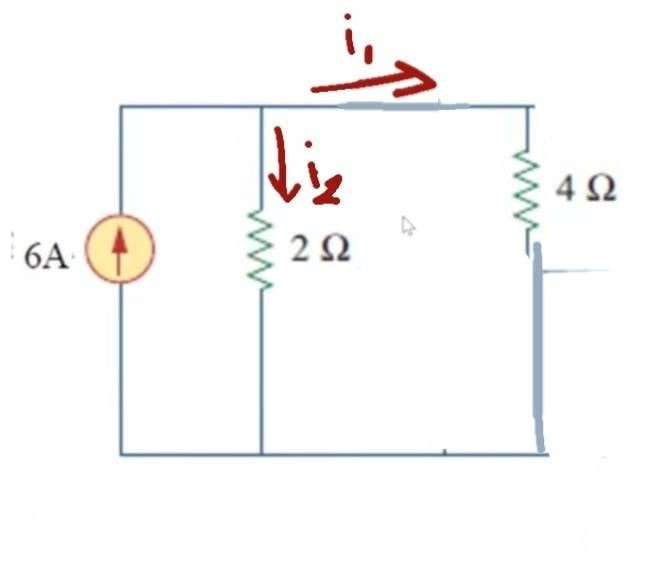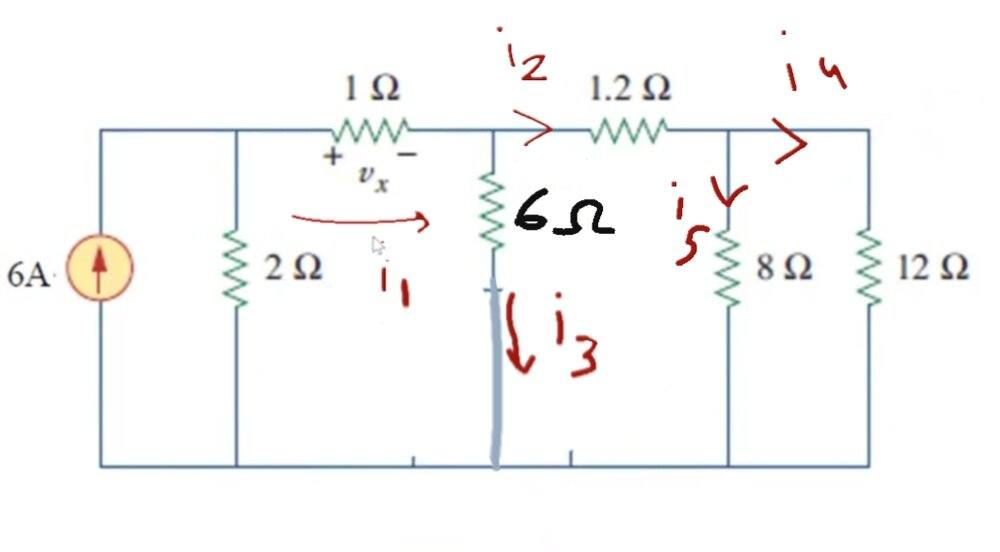# Help in Electric Circuit Analysis -- One current source and a resistor network

• Engineering
• Xiao Xiao
At first I didn't the same as in the second circuit, I used current division on the (1ohm and 2ohm resistors) then got the currents for other branches using the same method, but it was the wrong answer and the correct one was by doing like in the first circuit first, but I don't understand why I was wrong, and why it doesn't work for i1 like it does for the other currents. They're all connected to just two resistors.Edit: okay, wait, forget what is said, so I was wrong, the correct thing to do, using the second circuit in my previous reply, first Req=6 (for the 8||12=4.8 and withf

#### Xiao Xiao

Homework Statement
In the circuit shown, determine Vx and the power absorbed by the 12 ohm resistor.
Relevant Equations
We used Ohm's law and current division.

First we found Vx by making and Req which equals to 6ohm (the circuit will like like a branch with as shown in the second circuit) we find the current in the 4ohm resistor branch using current division.

And to find the power absorbed in 12 ohm resistor we need to find the value of current that passes through that branch (i4), using current dividor as shown in the third circuit. What I don't get is why did we put an Req to find i1, we had only two resistor branches involved, so why couldn't it have worked if we used Req with the 1ohm, but it worked to find the current in other branches (after making an Req for each wire(?)?

Edit: turns out we can't put two pictures or idk, anyway, we found an Req first, so the circuit would look like, the current with ameber branch, the two parallel resistor branches (2ohm and Req=4ohm) and we used that to find Vx.

Then we go back to the original circuit, we make Req=6ohm (using the branch with 4ohm, 3 ohm, 6ohm, resistors). If that helps imagining how it looks.o9

I'm only seeing one circuit diagram, but it sounds from your description that there are 3 total diagrams?

I'm only seeing one circuit diagram, but it sounds from your description that there are 3 total diagrams?
Yeah I noticed it didn't work, and tried to describe it with words instead.

It sounded like you had the right approach. Fold up (combine) all the resistors to the right of the Vx resistor, and find Vx. Then start un-folding them back to work your way to finding the voltage and current for the 12 Ohm resistor. Can you post your work on that?

It sounded like you had the right approach. Fold up (combine) all the resistors to the right of the Vx resistor, and find Vx. Then start un-folding them back to work your way to finding the voltage and current for the 12 Ohm resistor. Can you post your work on that?It sounded like you had the right approach. Fold up (combine) all the resistors to the right of the Vx resistor, and find Vx. Then start un-folding them back to work your way to finding the voltage and current for the 12 Ohm resistor. Can you post your work on that?
View attachment 281124
View attachment 281125View attachment 281124
At first I didn't the same as in the second circuit, I used current division on the (1ohm and 2ohm resistors) then got the currents for other branches using the same method, but it was the wrong answer and the correct one was by doing like in the first circuit first, but I don't understand why I was wrong, and why it doesn't work for i1 like it does for the other currents. They're all connected to just two resistors.

Edit: okay, wait, forget what is said, so I was wrong, the correct thing to do, using the second circuit in my previous reply, first Req=6 (for the 8||12=4.8 and with 1.2 ohm resister). Then we find i2, after that we unfold it back and find i4? So to use current division there has to be only two resistors that aren't connected to any other branch (the current doesn't get divided after its done going though them)?

Last edited: